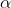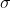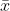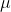Question

# The number of active coal mines in Utah has steadily decreased since 1960. However, peak production...

The number of active coal mines in Utah has steadily decreased since 1960. However, peak production occurred in 2001. One measure of mine productivity is tons/employee hour. In a random sample of 40 days, the mean tons/employee hour was 4.97. Assume σ = 1.16. Find a 95% confidence interval for the true mean tons/employee hour. (Round your answers to four decimal places.)

____________, _____________ tons/employee hour

Solution :

Given that,

Sample size = n = 40

Z/2 = 1.96

Margin of error = E = Z/2* (/n)

= 1.96 * (1.16 /40 )

= 0.3595

At 95% confidence interval estimate of the population mean is,- E <<+ E

4.97 - 0.3595 << 4.97 + 0.3595

4.6105 << 5.3295

4.6105 , 5.3295 tons/employee hour

#### Earn Coins

Coins can be redeemed for fabulous gifts.